# Pressure

Pressure is defined as force per unit area. It is usually more convenient to use pressure rather than force to describe the influences upon fluid behavior. The standard unit for pressure is the Pascal, which is a Newton per square meter.

For an object sitting on a surface, the force pressing on the surface is the weight of the object, but in different orientations it might have a different area in contact with the surface and therefore exert a different pressure.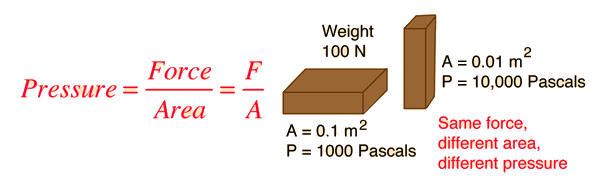### Pressure calculation.

There are many physical situations where pressure is the most important variable. If you are peeling an apple, then pressure is the key variable: if the knife is sharp, then the area of contact is small and you can peel with less force exerted on the blade. If you must get an injection, then pressure is the most important variable in getting the needle through your skin: it is better to have a sharp needle than a dull one since the smaller area of contact implies that less force is required to push the needle through the skin.

When you deal with the pressure of a liquid at rest, the medium is treated as a continuous distribution of matter. But when you deal with a gas pressure, it must be approached as an average pressure from molecular collisions with the walls.

Pressure in a fluid can be seen to be a measure of energy per unit volume by means of the definition of work. This energy is related to other forms of fluid energy by the Bernoulli equation.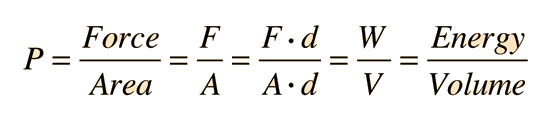Index

Pressure concepts

 HyperPhysics***** Mechanics ***** Fluids R Nave
Go Back

# Pressure as Energy Density

Pressure in a fluid may be considered to be a measure of energy per unit volume or energy density. For a force exerted on a fluid, this can be seen from the definition of pressure:The most obvious application is to the hydrostatic pressure of a fluid, where pressure can be used as energy density alongside kinetic energy density and potential energy density in the Bernoulli equation.

The other side of the coin is that energy densities from other causes can be conveniently expressed as an effective "pressure". For example, the energy density of solvent molecules which leads to osmosis is expressed as osmotic pressure. The energy density which keeps a star from collapsing is expressed as radiation pressure.

Index

Bernoulli concepts

 HyperPhysics***** Mechanics ***** Fluids R Nave
Go Back

# Fluid Kinetic Energy

The kinetic energy of a moving fluid is more useful in applications like the Bernoulli equation when it is expressed as kinetic energy per unit volume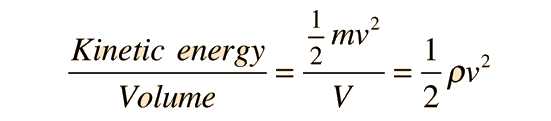When the kinetic energy is that of fluid under conditions of laminar flow through a tube, one must take into account the velocity profile to evaluate the kinetic energy. Across the cross-section of flow, the kinetic energy must be calculated using the average of the velocity squared, which is not the same as squaring the average velocity. Expressed in terms of the maximum velocity vm at the center of the flow, the kinetic energy is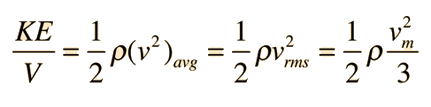Show more detail
Index

Bernoulli concepts

 HyperPhysics***** Mechanics ***** Fluids R Nave
Go Back

# Fluid Potential Energy

The potential energy of a moving fluid is more useful in applications like the Bernoulli equation when it is expressed as potential energy per unit volume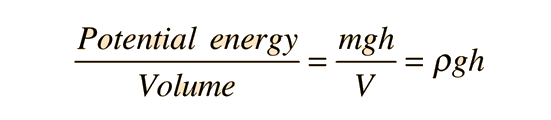The energy density of a fluid can be expressed in terms of this potential energy density along with kinetic energy density and fluid pressure.

Index

Bernoulli concepts

 HyperPhysics***** Mechanics ***** Fluids R Nave
Go Back